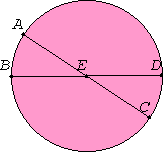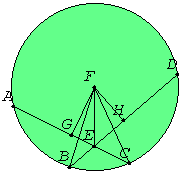# Proposition 35

If in a circle two straight lines cut one another, then the rectangle contained by the segments of the one equals the rectangle contained by the segments of the other.

For in the circle ABCD let the two straight lines AC and BD cut one another at the point E.I say that the rectangle AE by EC equals the rectangle DE by EB.

If now AC and BD are through the center, so that E is the center of the circle ABCD, it is manifest that, AE, EC, DE, and EB being equal, the rectangle AE by EC also equals the rectangle DE by EB.Next let AC and DB not be through the center. Take the center F let the center of ABCD. Draw FG and FH from F perpendicular to the straight lines AC and DB. Join FB, FC, and FE.

III.3

Then, since a straight line GF through the center cuts a straight line AC not through the center at right angles, it also bisects it, therefore AG equals GC.

II.5

Since, then, the straight line AC has been cut into equal parts at G and into unequal parts at E, the rectangle AE by EC together with the square on EG equals the square on GC.

Add the square on GF. Therefore the rectangle AE by EC plus the sum of the squares on GE and GF equals the sum of the squares on CG and GF.

I.47

But the square on FE equals the sum of the squares on EG and GF, and the square on FC equals the sum of the squares on CG and GF. Therefore the rectangle AE by EC plus the square on FE equals the square on FC.

And FC equals FB, therefore the rectangle AE by EC plus the square on EF equals the square on FB.

For the same reason, also, the rectangle DE by EB plus the square on FE equals the square on FB.

But the rectangle AE by EC plus the square on FE was also proved equal to the square on FB, therefore the rectangle AE by EC plus the square on FE equals the rectangle DE by EB plus the square on FE.

Subtract the square on FE from each. Therefore the remaining rectangle AE by EC equals the rectangle DE by EB.

Therefore if in a circle two straight lines cut one another, then the rectangle contained by the segments of the one equals the rectangle contained by the segments of the other.

Q.E.D.

## Guide

By means of proposition VI.16, the statement, “the rectangle AE by EC equals the rectangle DE by EB,” may be converted into one about ratios, namely, “AE : EB = DE : EC.

This proposition is not used in the rest of the Elements.